Win up to 100% scholarship on Aakash BYJU'S JEE/NEET courses with ABNAT Win up to 100% scholarship on Aakash BYJU'S JEE/NEET courses with ABNAT

# JEE Main 2018 Chemistry Paper With Solutions Shift 1 Jan 10

JEE Main 2018 Chemistry paper January 10 Shift 1 solutions are available on this page. Students are recommended to revise these solutions so that they can improve their problem-solving skills. Students can easily access these solutions and download them in PDF format for free. These solutions help to understand the difficulty level of questions from each chapter.
January 10 Shift 1 – Chemistry
1. Which of the following salts is the most basic in aqueous solution?
1) CH3COOK
2) FeCl3
3) Pb(CH3COO)2
4) Al(CN)3

CH3COOK + H2O -> CH3COOH + KOH (basic)

FeCl3 – Acidic solution

Al(CN)3 – Salt of weak acid and weak base

Pb(CH3COO)2 – Salt of weak acid and weak base

CH3COOK is salt of weak acid and strong base.

Hence solution of CH3COOK is basic.

2. Which of the following compounds will be suitable for Kjeldahl’s method for nitrogen estimation?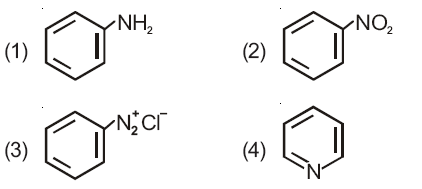Kjeldahl method is not applicable for compounds containing nitrogen in nitro, and azo groups and nitrogen in ring, as N of these compounds does not change to ammonium sulphate under these conditions. Hence only aniline can be used for estimation of nitrogen by Kjeldahl’s method.

3. Which of the following are Lewis acids?
1) AlCl3 and SiCl4
2) PH3 and SiCl4
3) BCl3 and AlCl3
4) PH3 and BCl3

BCl3 – electron deficient, incomplete octet

AlCl3 – electron deficient, incomplete octet

SiCl4 can accept lone pair of electron in d-orbital of silicon hence it can act as Lewis acid.

Although the most suitable answer is (3). However, both option (3) and (1) can be considered as correct answers.

Eg. Hydrolysis of SiCl4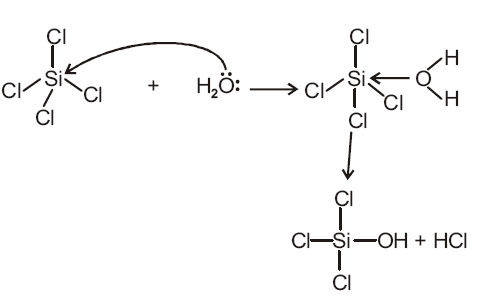4. Phenol on treatment with CO2 in the presence of NaOH followed by acidification produces compound X as the major product. X on treatment with (CH3CO)2O in the presence of catalytic amount of H2SO4 produces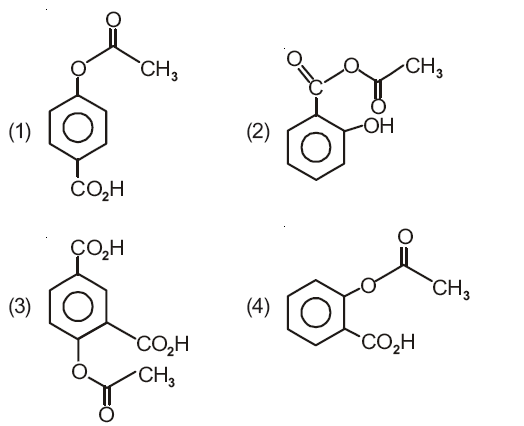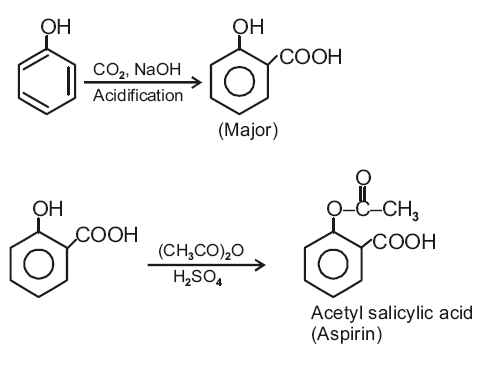5. An alkali is titrated against an acid with methyl orange as indicator, which of the following is a correct combination?

 Base Acid End point 1. Strong Strong Pinkish red to yellow 2. Weak Strong Yellow to Pinkish red 3. Strong Strong Pink to colourless 4. Weak Strong Colourless to pink

The pH range of methyl orange is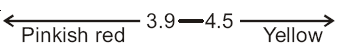Weak base is having pH greater than 7. When methyl orange is added to weak base solution, the solution becomes yellow. This solution is titrated by strong acid and at the end point pH will be less than 3.1. Therefore solution becomes pinkish red.

6. An aqueous solution contain 0.10 M H2S and 0.20 M HCl. If the equilibrium constant for the formation of HS from H2S is 1.0×10-7 and that of S2- from HS ions is 1.2×10-13 then the concentration of S2- ions in aqueous solution is
1) 3×10-20
2) 6×10-21
3) 5×10-19
4) 5×10-8

$$\begin{array}{l}H_{2}S\rightleftharpoons 2H^{+}+S^{2-}\end{array}$$

Ka1.Ka2 = Keq

$$\begin{array}{l}\frac{{[H^{+}]^{2}[S^{2-}]}}{[H_{2}S]} = 1\times 10^{-7}\times 1.2\times 10^{-13}\end{array}$$
$$\begin{array}{l}\frac{{[0.2]^{2}[S^{2-}]}}{[0.1]} = 1.2\times 10^{-20}\end{array}$$
[S2-] = 3×10-20

7. The combustion of benzene (l) gives CO2(g) and H2O (l). Given that heat of combustion of benzene at constant volume is -3263.9 kJ mol -1 at 250C ; heat of combustion (in kJ/mol) of benzene at constant pressure will be (R = 8.314 JK-1 mol-1)
1) -452.46
2) 3260
3) -3267.6
4) 4152.6

C6H6 (l) + (15/2)O2 (g) -> 6CO2 (g) + 3H2O (l)

Δng = 6-(15/2)

= -3/2

ΔH = ΔU +ΔngRT

= -3263.9 +(-3/2)×8.314×298×10-3

= -3263.9 + (-3.71)

= -3267.6 kJ mol-1

8. The compound that does not produce nitrogen gas by the thermal decomposition is
1) (NH4)2Cr2O7
2) NH4NO2
3) (NH4)2SO4
4) Ba(N3)2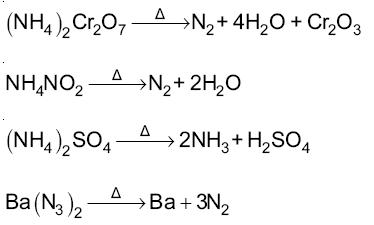Among all the given compounds, only (NH4)2SO4 do not form dinitrogen on heating, it produces ammonia gas.

9. How long (approximate) should water be electrolysed by passing through 100 amperes current so that the oxygen released can completely burn 27.66 g of diborane? (Atomic weight of B = 10.8 u)
1) 0.8 hours
2) 3.2 hours
3) 1.6 hours
4) 6.4 hours

B2H6 +3O2 -> B2O3 +3H2O

27.66 of B2H6 = 1 mole of B2H6 which requires three moles of oxygen (O2) for complete burning

6H2O -> 6H2 +3O2 (On electrolysis)

Number of faradays = 12 = amount of charge

12×96500 = i×t

12×96500 = 100×t

t = 12×96500/100 sec

t = 12×96500/(100×3600) hour

t = 3.2 hours

10. Total number of lone pair of electrons in I3 ion is
1) 6
2) 9
3) 12
4) 3

Structure of I3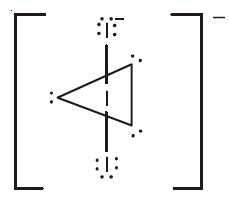Number of lone pairs in I3 is 9.

11. When metal ‘M’ is treated with NaOH, a white gelatinous precipitate ‘X’ is obtained, which is soluble in excess of NaOH. Compound ‘X’ when heated strongly gives an oxide which is used in chromatography as an adsorbent. The metal ‘M’ is
1) Ca
2) Al
3) Fe
4) Zn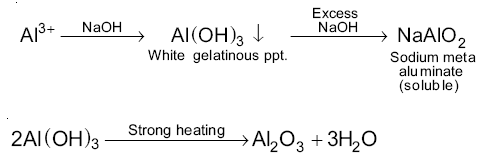Al2O3 is used in column chromatography.

12. According to molecular orbital theory, which of the following will not be a viable molecule?
1) He2+
2) H2
3) H22-
4) He22+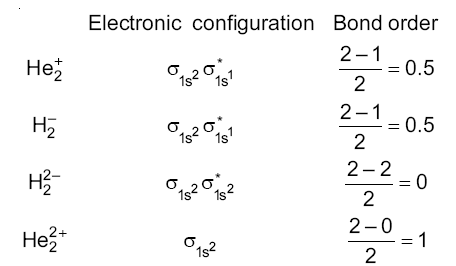Molecule having zero bond order will not be a viable molecule.

13. The correct order of basicity of the following compound is :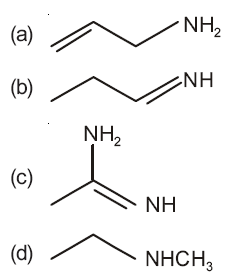1) (b) < (a) < (c) < (d)
2) (b) < (a) < (d) < (c)
3) (d) < (b) < (a) < (c)
4) (a) < (b) < (c) < (d)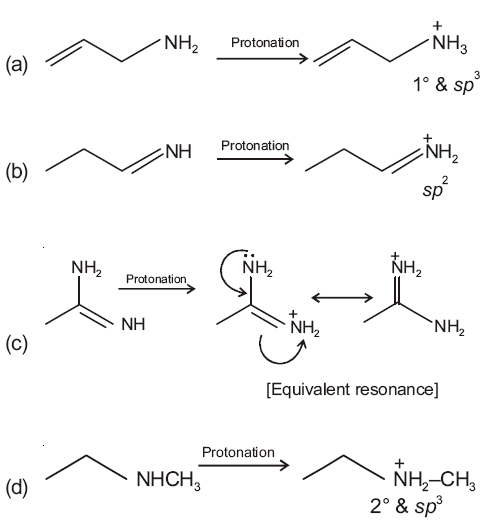Correct order of basicity is: b < a < d < c

14. Which type of ‘defect’ has the presence of cations in the interstitial sites?
1) Vacancy defect
2) Frenkel defect
3) Metal deficiency defect
4) Schottky defect

In Frenkel defect, cation is dislocated from its normal lattice site to an interstitial site.

15. Which of the following compounds contain(s) no covalent bond(s)?

KCl, PH3, O2, B2H6, H2SO4

1) KCl, H2SO4
2) KCl
3) KCl, B2H6
4) KCl, B2H6, PH3

KCl – Ionic bond between K+ and Cl

PH3– Covalent bond between P and H

O2 – Covalent bond between O atoms

B2H6 – Covalent bond between B and H atoms

H2SO4– Covalent bond between S and O and also between O and H.

Compound having no covalent bonds is KCl only.

16. The oxidation states of Cr in [Cr(H2O)6]Cl3, [Cr(C6H6)2] and K2[Cr(CN)2(O)2(O2)(NH3)] respectively are
1) +3, +2 and +4
2) +3, 0 and +6
3) +3, 0 and +4
4) +3, +4 and +6

[Cr(H2O)6]Cl3 ⇒ x+0×6-1×3 = 0

x = +3

[Cr(C6H6)2] ⇒ x+2×0 = 0

x = 0

K2[Cr(CN)2(O)2(O2)(NH3)] ⇒ 1×2+x-1×2-2-2×1 = 0

⇒ x-6 = 0

So x = +6

17. Hydrogen peroxide oxidises [Fe(CN)6]4- to [Fe(CN)6]3- in acidic medium but reduces [Fe(CN)6]3- to [Fe(CN)6]4- in alkaline medium. The other products formed are, respectively.
1) (H2O+O2) and (H2O+OH)
2) H2O and (H2O+O2)
3) H2O and (H2O+ OH)
4) (H2O+O2) and H2O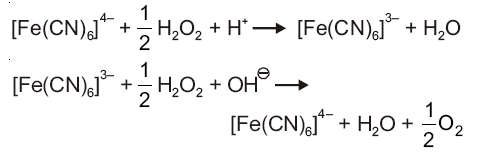18. Glucose on prolonged heating with HI gives
1) 1-Hexene
2) Hexanoic acid
3) 6-iodohexanal
4) n-Hexane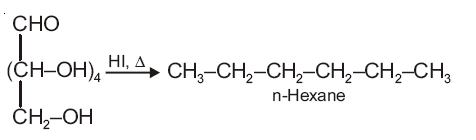19. The predominant form of histamine present in human blood is (pKa Histidine = 6.0)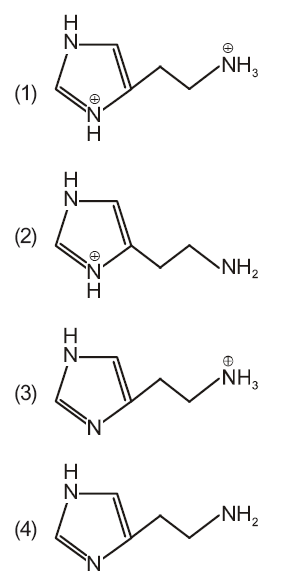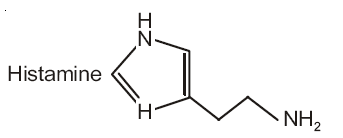At pH (7.4) major form of histamine is protonated at primary amine.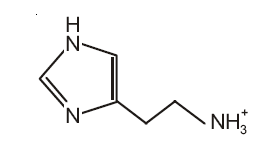20. The recommended concentration of fluoride ion in drinking water is up to 1 ppm as fluoride ion is required to make teeth enamel harder by converting [3Ca3(PO4)2.Ca(OH)2] to
1) [3Ca(F)2.Ca(OH)2]
2) [3Ca3(PO4)2.CaF2]
3) [3{Ca(OH)2}.Ca(F)2]
4) [CaF2]

F ions make the teeth enamel harder by converting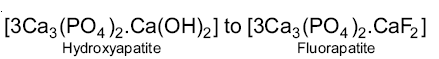21. Consider the following reaction and statements

[Co(NH3)4Br2]+ + Br [Co(NH3)3Br3]+NH3

(I) Two isomers are produced if the reactant complex ion is a cis-isomer

(II) Two isomers are produced if the reactant complex ion is a trans-isomer.

(III) Only one isomer is produced if the reactant complex ion is a trans-isomer.

(IV) Only one isomer is produced if the reactant complex ion is a cis-isomer.

The correct statements are:

1) (I) and (III)
2) (III) and (IV)
3) (II) and (IV)
4) (I) and (II)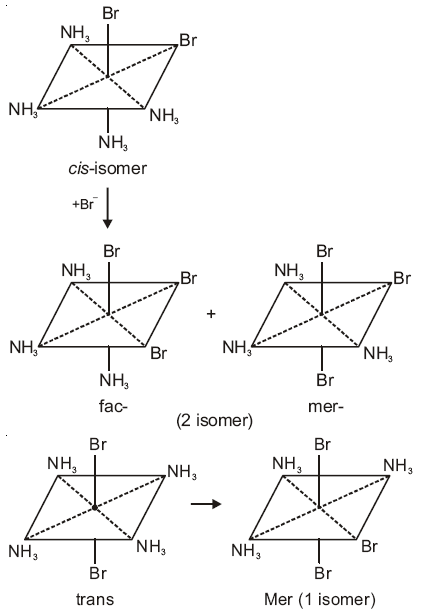So option (1) is correct.

22. The trans-alkenes are formed by the reduction of alkynes with
1) NaBH4
2) Na/liq. NH3
3) Sn – HCl
4) H2-Pd/C, BaSO4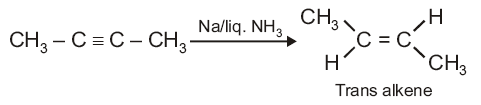So, option (2) is correct.

23. The ratio of mass percent of C and H of an organic compound (CXHYOZ) is 6 : 1. If one molecule of the above compound (CXHYOZ) contains half as much oxygen as required to burn one molecule of compound CXHY completely to CO2 and H2O. The empirical formula of compound CXHYOZ is
1) C2H4O
2) C3H4O2
3) C2H4O3
4) C3H6O3

 Element Relative mass Relative mole Simplest whole number ratio C 6 6/12 = 0.5 1 H 1 1/1 = 1 2

So, X = 1, Y = 2

Equation for combustion of CXHY

CXHY +(X+Y/4)O2 -> XCO2 +(Y/2)H2O

Oxygen atoms required = 2(X+Y/4)

Given 2(X+Y/4) = 2Z

⇒(1+2/4) = Z

⇒ Z = 1.5

Molecule can be written as CXHYOZ

⇒ C1H2O3/2

⇒ C2H4O3

24. Phenol reacts with methyl chloroformate in the presence of NaOH to form product A. A reacts with Br2 to form product B. A and B are respectively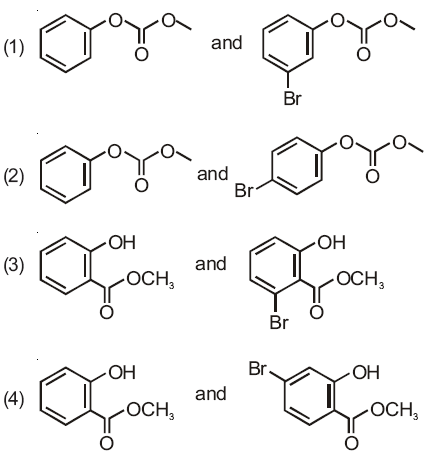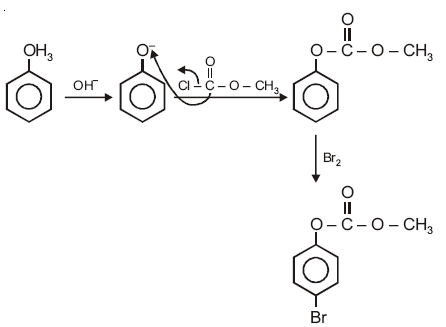25. The major product of the following reaction is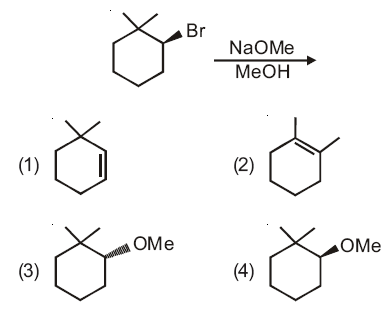CH3O is a strong base and strong nucleophile, so favourable condition is SN2/E2.

Given alkyl halide is 20 and βC’s are 40 and 20 , so sufficiently hindered, therefore, E2 dominates over SN2.

Also, polarity of CH3OH (solvent) is not as high as H2O so E1 is also dominated by E2.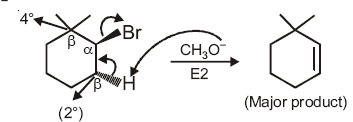26. Which of the following lines correctly show the temperature dependence of equilibrium constant K, for an exothermic reaction?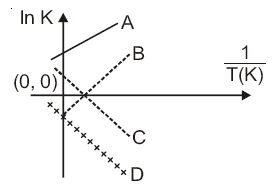1) B and C
2) C and D
3) A and D
4) A and B

Equilibrium constant K =

$$\begin{array}{l}(\frac{A_{f}}{A_{b}})e^{-\Delta H^{0}/RT}\end{array}$$

ln K = ln (Af/Ab) – (ΔH0/R)(1/T)

y = c+mx

comparing with equation of straight line,

slope = -ΔH0/R

Since, reaction is exothermic, ΔH0 = -ve, therefore,

slope = +ve.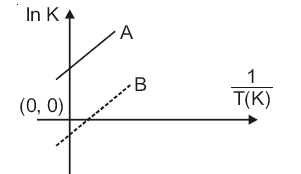27. The major product formed in the following reaction is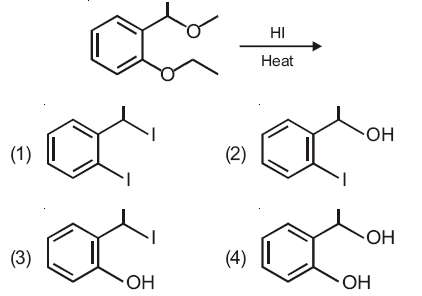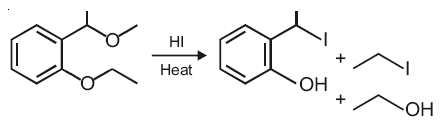28. An aqueous solution contains an unknown concentration of Ba2+ . When 50 mL of a 1 M solution of Na2SO4 is added, BaSO4 just begins to precipitate. The final volume is 500 mL. The solubility product of BaSO4 is 1×10–10 . What is original concentration of Ba2+ ?
1) 2 ×10-9 M
2) 1.1×10-9 M
3) 1.0×10-9 M
4) 5 ×10-9 M

Final concentration of [SO4] = 50×1/500 = 0.1M

Ksp of BaSO4,

[Ba2+] [SO42-] = 1×10-10

[Ba2+] [0.1] = 10-10/0.1 = 10-9 M

Concentration of Ba2+ in final solution = 10-9 M

Concentration of Ba2+ in original solution.

M1V1 = M2V2

M1(500-50) = 10-9(500)

M1 = 1.11×10-9 M

29. At 5180 C, the rate of decomposition of a sample of gaseous acetaldehyde, initially at a pressure of 363 torr, was 1.00 torr s–1 when 5% had reacted and 0.5 torr s–1 when 33% had reacted. The order of the reaction is
1) 3
2) 1
3) 0
4) 2

Assume the order of reaction with respect to acetaldehyde is x.

Condition 1:

Rate = k[CH3CHO]x

1 = k[363×0.95]x

1 = k[344.85]x ..(i)

Condition 2:

0.5 = k[363×0.67]x

0.5 = k[243.21]x ..(ii)

Divide equation (i) by (ii),

1/0.5 = (344.85/243.21)x

⇒2 = 1.414x

⇒ x = 2

30. For 1 molal aqueous solution of the following compounds, which one will show the highest freezing point?
1) [Co(H2O)5Cl]Cl2. H2O
2) [Co(H2O)4Cl2 ]Cl. 2H2O
3) [Co(H2O)3Cl3]. 3H2O
4) [Co(H2O)6 ]Cl3

The solution which shows maximum freezing point must have minimum number of solute particles.

(1) [Co(H2O)5Cl]Cl2. H2O ->[Co(H2O)5Cl]2+ +2Cl, i = 3

(2) [Co(H2O)4Cl2 ]Cl. 2H2O -> [Co(H2O)4Cl2]+ +Cl, i = 2

(3) [Co(H2O)3Cl3]. 3H2O -> [Co(H2O)3Cl3 ] , i = 1

(4) [Co(H2O)6 ]Cl3 -> [Co(H2O)6 ]3+ +3Cl , i = 4

So, solution of 1 molal [Co(H2O)3Cl3]. 3H2O will have minimum number of particles in aqueous state.